# SAT Math : How to find the length of the hypotenuse of a right triangle : Pythagorean Theorem

## Example Questions

### Example Question #481 : Psat Mathematics

Given the right triangle in the diagram, what is the length of the hypotenuse?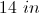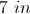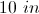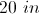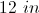Explanation:

To find the length of the hypotenuse use the Pythagorean Theorem: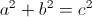Where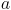andare the legs of the triangle, and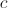is the hypotenuse.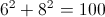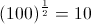The hypotenuse is 10 inches long.

### Example Question #41 : Triangles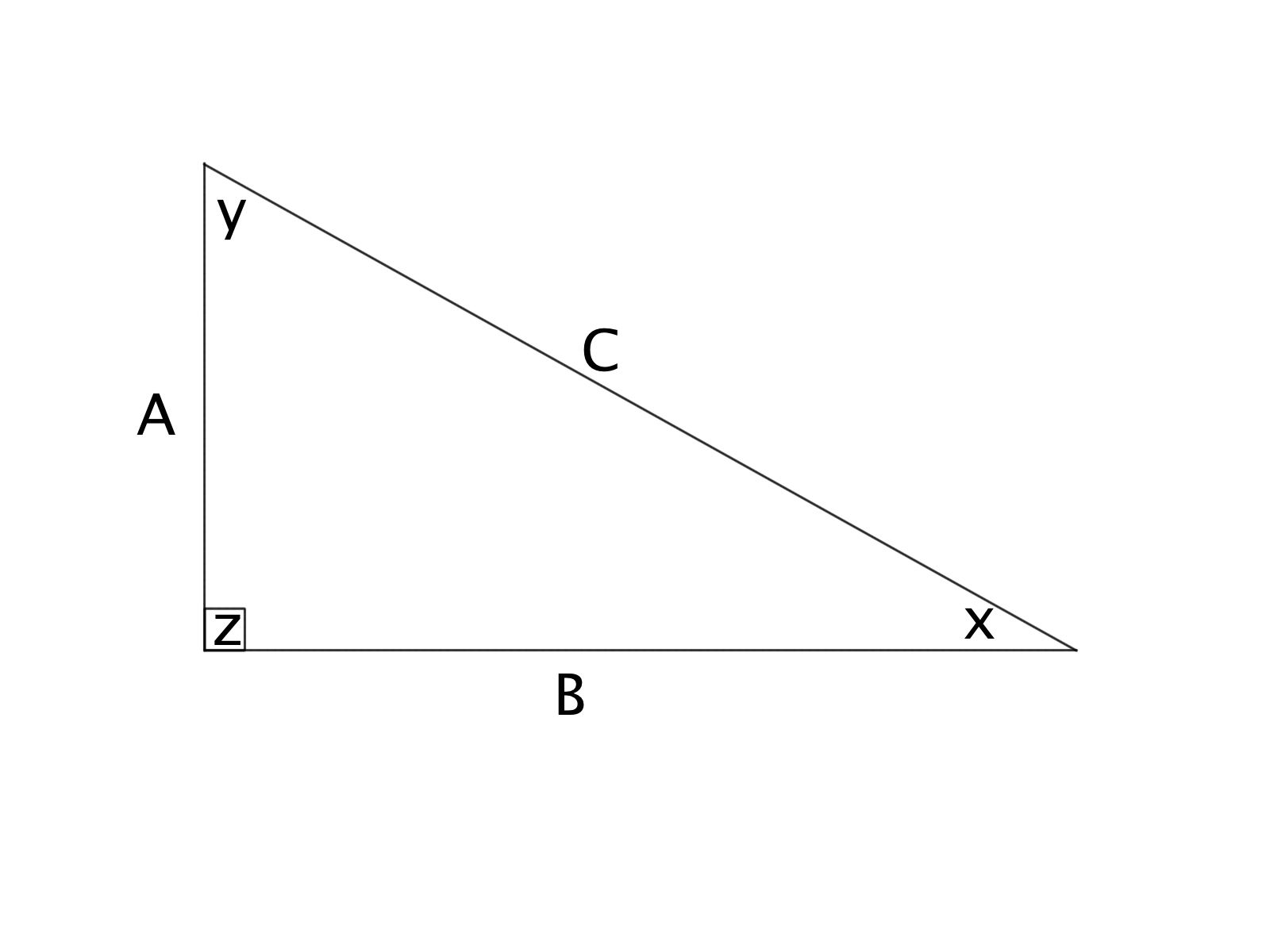Triangle ABC is a right triangle. If the length of side A = 3 inches and C = 5 inches, what is the length of side B?

6 inches

4 inches

1/2 inches

1 inches

4.5 inches

4 inches

Explanation:

Using the Pythagorean Theorem, we know that.

This gives: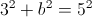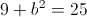Subtracting 9 from both sides of the equation gives: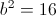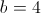inches### Example Question #64 : Right TrianglesTriangle ABC is a right triangle. If the length of side A = 8 inches and B = 11 inches, find the length of the hypoteneuse (to the nearest tenth).

14.2 inches

184 inches

185 inches

13.6 inches

13.7 inches

13.6 inches

Explanation:

Using the Pythagrean Theorem, we know that.

This tells us: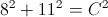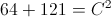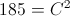Taking the square root of both sides, we find that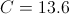inches

### Example Question #21 : How To Find The Length Of The Hypotenuse Of A Right Triangle : Pythagorean TheoremGiven:

A = 6 feet

B = 9 feet

What is the length of the hypoteneuse of the triangle (to the nearest tenth)?

10.1 feet

10.2 feet

10.6 feet

10.8 feet

10.5 feet

10.8 feet

Explanation:

Using the Pythagrean Theorem, we know that.

This tells us: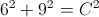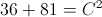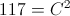Taking the square root of both sides, we find that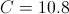### Example Question #22 : How To Find The Length Of The Hypotenuse Of A Right Triangle : Pythagorean TheoremGiven:

A = 2 miles

B = 3 miles

What is the length of the hypoteneuse of triangle ABC, to the nearest tenth?

3.5 miles

3.4 miles

3.7 miles

3.2 miles

3.6 miles

3.6 miles

Explanation:

Using the Pythagrean Theorem, we know that.

This tells us: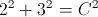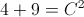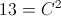Taking the square root of both sides, we find that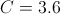### Example Question #67 : Right Triangles

Given that two sides of a right triangle measure 2 feet and 3 feet, respectively, with a hypoteneuse of x, what is the perimeter of this right triangle (to the nearest tenth)?

8.6 feet

9.4 feet

3.6 feet

18 feet

6.4 feet

8.6 feet

Explanation:

Using the Pythagrean Theorem, we know that.

This tells us:Taking the square root of both sides, we find thatTo find the perimeter, we add the side lengths together, which gives us that the perimeter is: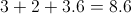### Example Question #101 : Geometry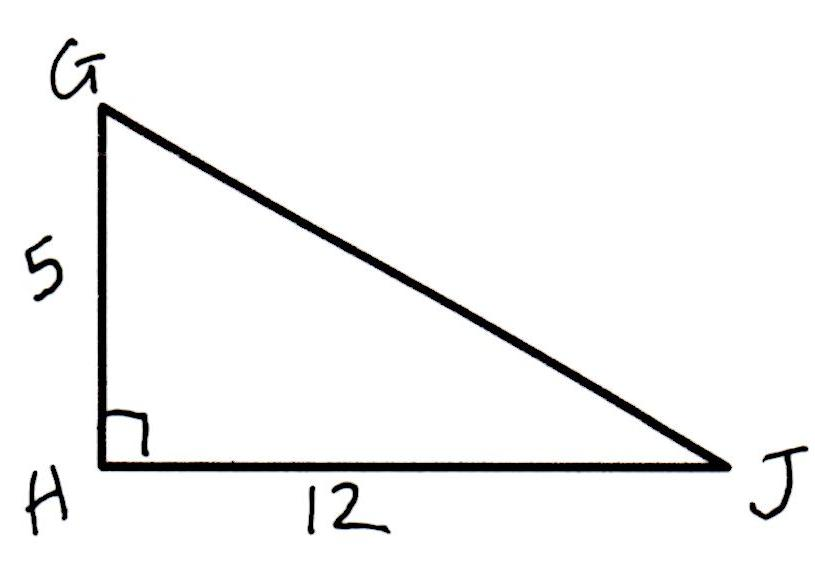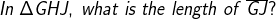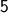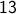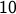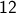Explanation: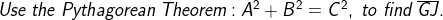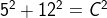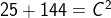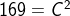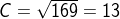### Example Question #23 : How To Find The Length Of The Hypotenuse Of A Right Triangle : Pythagorean Theorem

Kathy and Jill are travelling from their home to the same destination. Kathy travels due east and then after travelling 6 miles turns and travels 8 miles due north. Jill travels directly from her home to the destination. How miles does Jill travel?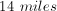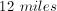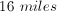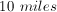Explanation:

Kathy's path traces the outline of a right triangle with legs of 6 and 8. By using the Pythagorean Theorem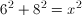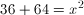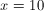miles

### Example Question #69 : Right Triangles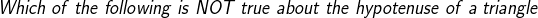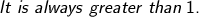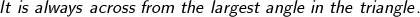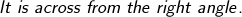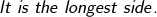Explanation: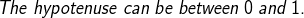### Example Question #24 : How To Find The Length Of The Hypotenuse Of A Right Triangle : Pythagorean Theorem

In order to get to work, Jeff leaves home and drives 4 miles due north, then 3 miles due east, followed by 6 miles due north and, finally, 7 miles due east.  What is the straight line distance from Jeff’s work to his home?

10√2

2√5

15

11

6√2